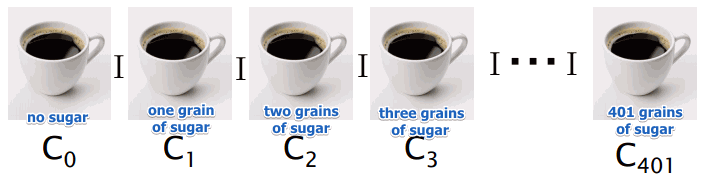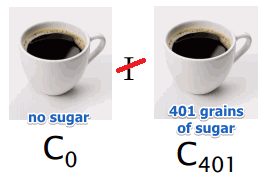# ML Wiki

## Luce's Coffee Cups

This is a thought experiment that shows that the Indifference relation is not transitive

Suppose we have 402 cups of coffee:

• $c_0$ with 0 grains of coffee
• $c_1$ with 1 grain of coffee, $c_0 \ I \ c_1$
• $c_2$ with 2 grains of coffee, $c_1 \ I \ c_2$
• ...
• $c_{401}$ with 401 grain of coffee, $c_{400} \ I \ c_{401}$
•But we cannot say that there's no difference in $c_0$ and $c_{401}$

• $c_0 \ \not I \ c_{401}$
•It shows that $I$ is not transitive:

• $a \ I \ b, b \ I \ c \not \Rightarrow a \ I \ c$

The reason for it is that there's a sensitivity threshold that is not taken into account